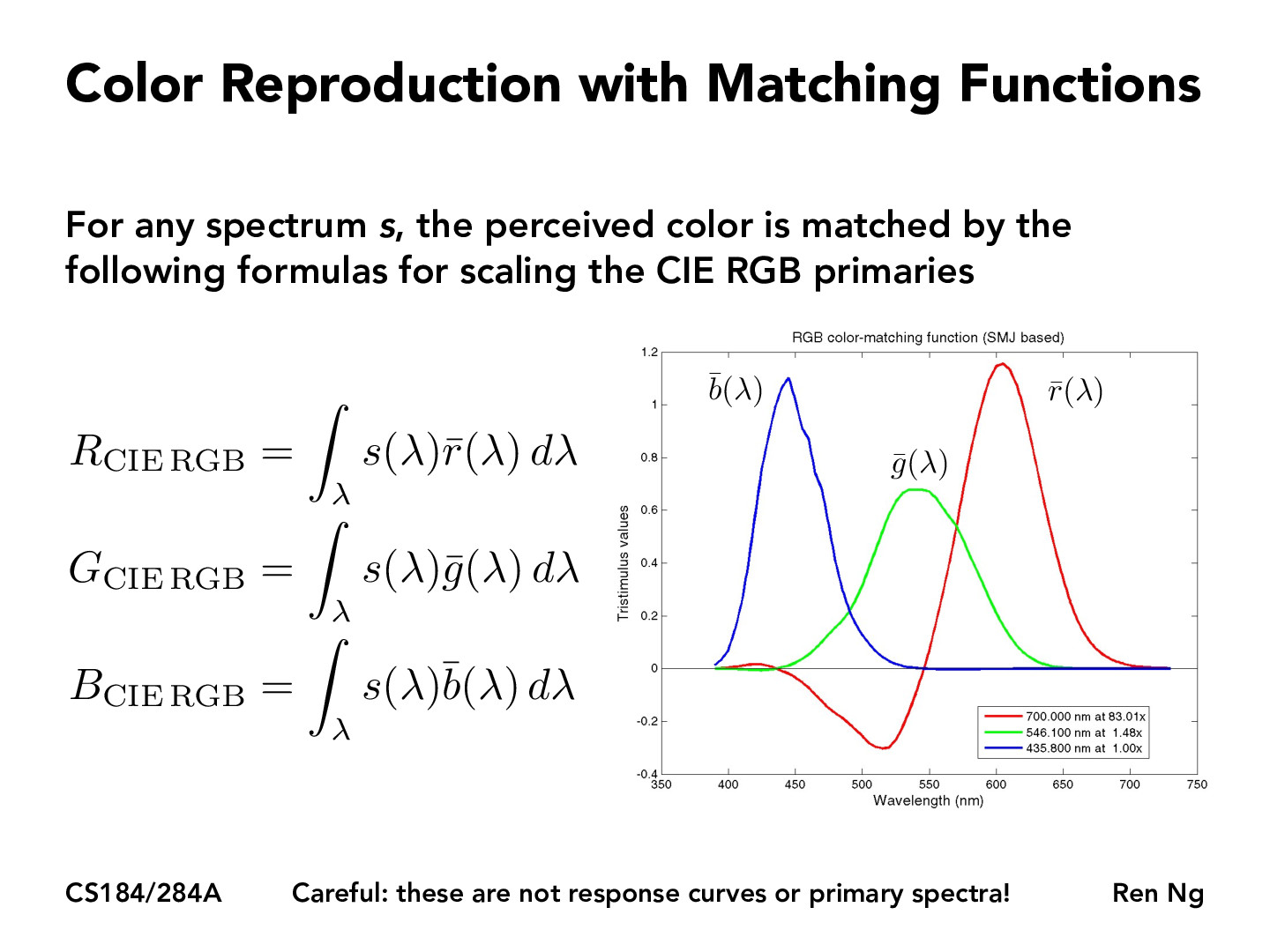You are viewing the course site for a past offering of this course. The current offering may be found here.Lecture 19: Introduction to Color Science (53)Michael-hsiu

The curves kind of remind me of Hermite polynomials, in that the ""B" curve has a hump for low wavelengths, while the "R" curve has a hump for higher wavelengths. Are they somehow related?Carpetfizz

From my understanding, if we are to draw a vertical line $x = \lambda$ for some wavelength $\lambda$, then that wavelength can be generated by adding the $\bar{b}(\lambda) + \bar{g}(\lambda) + \bar{r}(\lambda)$ that the line $x = \lambda$ intersects.

So, are the equations on the left trying to make the "opposite" statement, about how we can construct the primary colors using the full spectrum - instead of how we can construct a color at some arbitrary wavelength using the primary colors?amandaawan

From the above response, from my understanding, I would say that the colors in s(lambda) is not made simply from the three wavelengths of b, g, and r, but those are the wavelengths that we care about. Thus, adding numbers up at a certain wavelength wouldn't do that.

You must be enrolled in the course to comment